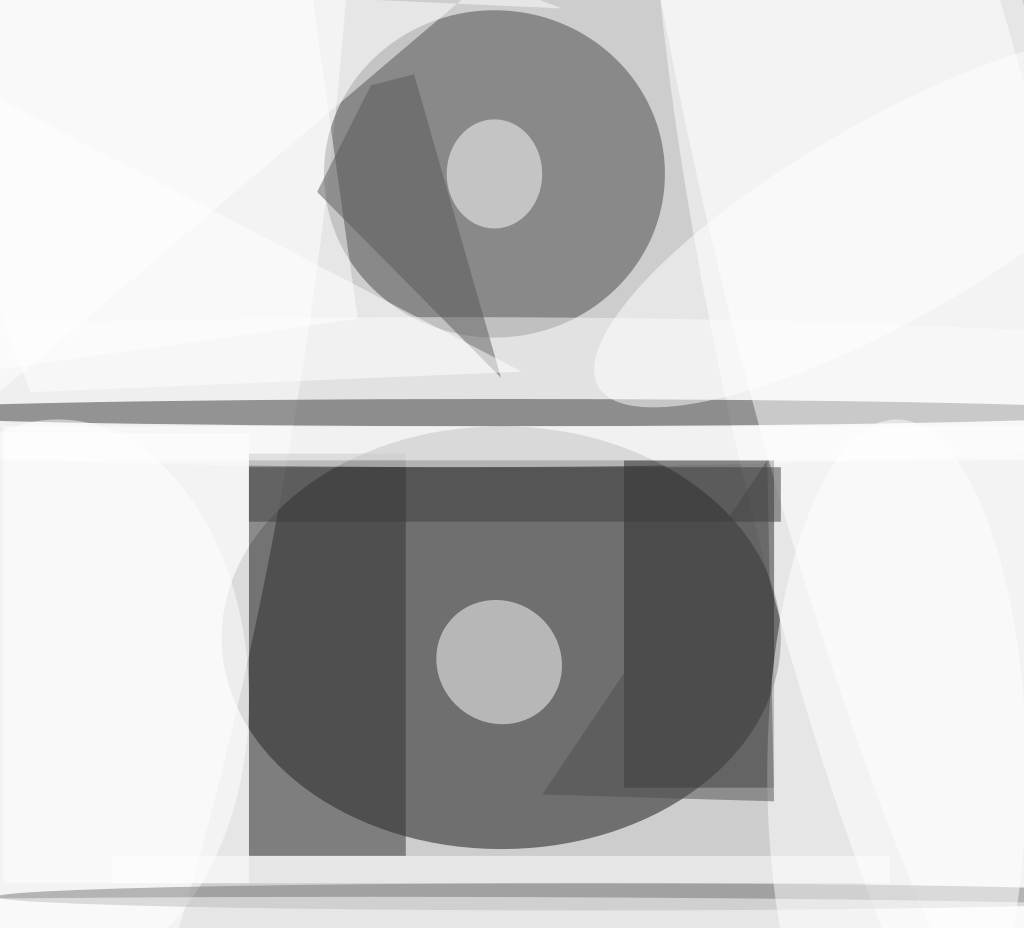Figure 1 depicts an apparatus that simultaneously and synchronously records magnified ordinary top-view video images and laser-shadowgraph video images of a sessile drop. The real-time values of contact angle and rate of evaporation of the drop as functions of time can be calculated from the apparent diameters of the drop in the sequences of the images. In addition, the shadowgraphs contain flow patterns indicative of thermocapillary convection (if any) within the drop. These time-dependent parameters and flow patterns are important for understanding the physical processes involved in the spreading of evaporating liquids in such diverse technological applications as coating (including painting), film cooling, processing of materials, lubrication, and boiling. Study of the spreading of drops can also contribute to understanding of the spreading of biological cells.Figure 1. The Time-Dependent Diameters d and D measured in images acquired by cameras 1 and 2 can be used to calculate the contact angle and rate of evaporation of the drop in the test section.

The apparatus includes a combination light source of a collimated white-light beam and a collimated laser beam (omitted from the figure), which are used to observe the ordinary top-view images and the shadowgraphs, respectively. Charge-coupled-device (CCD) camera 1 acquires the ordinary top-view video images, while CCD camera 2 acquires the shadowgraphs. The drop acts as a plano-convex lens, focusing the laser beam to a shadowgram on the projection screen in front of CCD camera 2.

The equations for calculating the contact angle and rate of evaporation of the drop are readily derived, starting from the basic equation for the focal length of a plano-convex lens with the drop size. The derivation is simplified by invoking the assumptions that (1) the mass of the drop is sufficiently small that its shape closely approximates a section of a sphere and (2) the drop is thin enough that its focusing action can be represented adequately by the thin-lens approximation. Omitting intermediate steps of the derivation for the sake of brevity, the results are the following:Figure 2. These Images Were Acquired Simultaneously, by use of the apparatus of Figure 1, during observation of an evaporating sessile drop of trichlorotrifluoroethane.

The time-dependent radius of curvature of the spherical drop surface is given by

R(t) = (n-1)(s+p) d(t)

d(t) + D(t)

where n is the index of refraction of the liquid in the drop, s and p are constant dimensions indicated in the figure, d is the time-dependent diameter of the drop as measured in the ordinary image acquired by CCD camera 1, and D is the time-dependent diameter of the shadowgram as measured in the image acquired by CCD camera 2 (see Figure 2).

The time-dependent contact angle is given by

θ(t) = arcsin d(t)

2R(t)

The rate of change of volume of the drop (the negative of the rate of evaporation) is given by

V= 2πhh(R - h/3) + πh2(R - h/3)

where

h = R - √ R2 - d2 / 4

and the overdot signifies differentiation with respect to time.

This work was done by David F. Chao of Glenn Research Center and Nengli Zhang of Ohio Aerospace Institute. For further information, access the Technical Support Package (TSP) free on-line at www.nasatech.com/tsp  under the Test and Measurement category.

Inquiries concerning rights for the commercial use of this invention should be addressed to

###### NASA Glenn Research CenterCommercial Technology OfficeAttn: Steve FedorMail Stop 4–821000 Brookpark RoadClevelandOhio 44135.

Refer to LEW-17075.